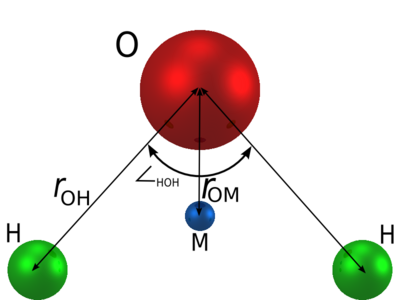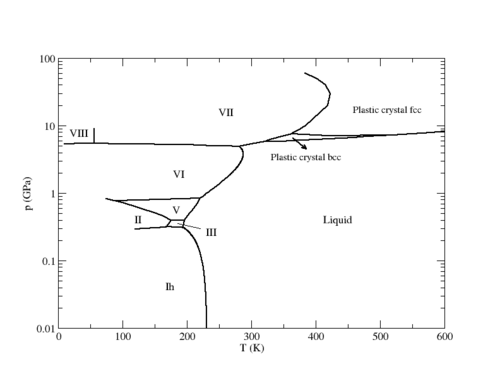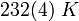2022: SklogWiki celebrates 15 years on-line

TIP4P model of water

(Redirected from TIP4P)

The TIP4P model  is a rigid planar four-site interaction potential for water, having a similar geometry to the Bernal and Fowler model, which was proposed in 1933.

Parameters

The TIP4P model consists of a Lennard-Jones site for the oxygen atom, and three charge sites.$r_{\mathrm {OH}}$ (Å)$\angle$HOH , deg$\sigma$ (Å)$\epsilon/k$ (K) q(O) (e) q(H) (e) q(M) (e)$r_{\mathrm {OM}}$ (Å) 0.9572 104.52 3.154 78.0 0 0.52 -2q(H) 0.15

Phase diagram

The following is the phase diagram of the TIP4P model in the pressure-temperature plane (adapted from Fig. 11 of ).Plastic crystal phases

Recent simulations have demonstrated the existence of plastic crystal phases for the TIP4P model .

Melting point

The melting point ice Ih-water at 1 bar is$232(4)~K$.

Shear viscosity

The shear viscosity for the TIP4P model is 0.494 mPa.s at 298 K and 1 bar  (experimental value 0.896 mPa.s ).

Virial coefficients

The second virial coefficient has been calculated by Chialvo et al .